Courses

# Logic Gates Class 12 Notes | EduRev

## Class 12 : Logic Gates Class 12 Notes | EduRev

The document Logic Gates Class 12 Notes | EduRev is a part of Class 12 category.
All you need of Class 12 at this link: Class 12

#### DiGITAL ELECTRONICS

Analogue Signal:

If the voltage (or) current in a circuit is a continuous function of time, then we call such signals as analog signals.

Example: Sinusoidal signals

Digital Signal:

Some voltage signals are not continuous and they are discrete pulses of only two voltage levels either zero (or) some constant value of voltage. Such signals are called digital signals.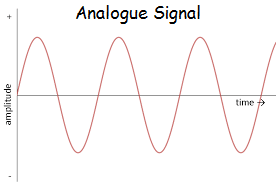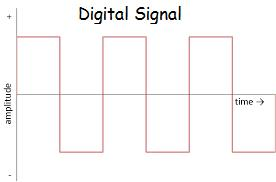#### lOGIC GATES

• A Logic Gate is a digital circuit that follows a certain logical relationship between the input and the output voltages. They are called as "gates" because they control the flow of information.
• Basically there are five common logic gates. They are NOT, AND, OR, NAND, NOR gates.
• Each logic gate is indicated by a symbol and it's function is defined by a truth table that shows all the possible input logic level combinations with their respective output logic levels.
• These logic gates are used in semiconductor devices.

NOT GATE

• It has only one input and one output.
• Logic diagram :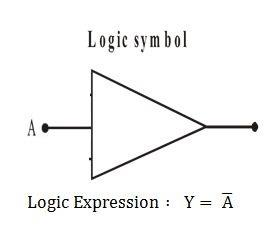Truth table:

 Input (A) Output (Y) 0 1 1 0

OR GATE

• It has two inputs and one output.
• Logic Diagram :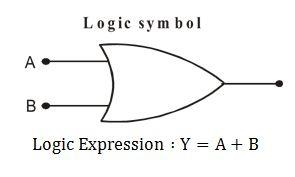• Truth table :
 Input (A) Input (B) Output (Y) 0 0 0 0 1 1 1 0 1 1 1 1
• A + 0 = A
• A + 1 = A
• A + A = 1
• A + Ã (not A) = 1

AND GATE

• It has two inputs and one output.
• Logic Diagram :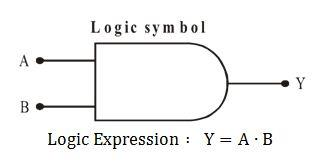• Truth Table :
 Input (A) Input (B) Output (Y) 0 0 0 0 1 0 1 0 0 1 1 1
• A • 0 = A • Ã = 0
• A • 1 = 1
• A • A = A
• A(B + C) = AB + AC = (A + B)(A + C)       (since A • A = A)
• A + AB = A(A + B) = A
• A + ÃB = A + B
• A(Ã + B) = AB

NOR GATE

• It is a combination of OR gate and followed by a NOT gate.
• Output of this gate is inverse of a OR gate.
• Logic Diagram :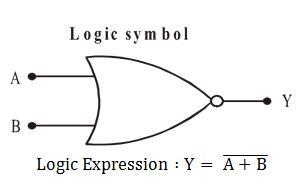• Truth Table :
 Input (A) Input (B) Output (Y) 0 0 1 0 1 0 1 0 0 1 1 0

NAND GATE

• It is a combination of AND gate and followed by a NOT gate.
• Output of this gate is inverse of the output of AND gate.
• Logic Diagram :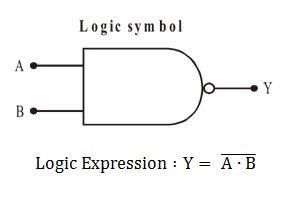• Truth Table :
 Input (A) Input (B) Output (Y) 0 0 1 0 1 1 1 0 1 1 1 0

Note: NAND gate and NOR gates are called Basic Building Blocks of digital electronic circuits (or) Universal Gates because any one of them alone can be used to construct any type of logic gate.

DE-MORGAN THEOREMS

This theorem is very useful in solving complicated circuits by converting them into simpler circuits.

•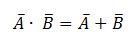•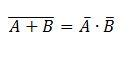Lastly there is another special type of logic gate called as the XNOR gate.

XNOR GATE

• Logic Diagram :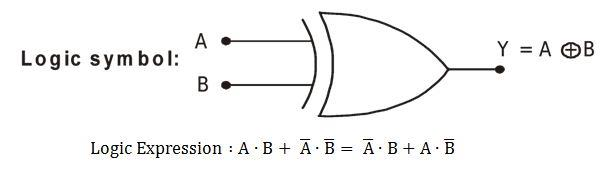• Truth Table :

 Input (A) Input (B) Output (Y) 0 0 1 0 1 0 1 0 0 1 1 1

Points To Remember:

• The basic concept of digital circuit has been provided by George Boole.
•  Claude Shannon established an analogy between function of mechanical switches and Boolean algebra.
• Series combination of switches is equivalent to AND logic operation.
• Parallel combination of switches is equivalent to OR logic operation.
• NOT logic operation is performed on a single variable. That’s why it is called unary operation.
• AND, OR and NOT logic operations follow closure property, i.e., input as well as output are in either of the binary states.
• NAND and NOR gates are universal gates.
• If the logic gate is changed from positive to negative or vice-versa; AND changes into OR, OR changes into AND, NAND changes into NOR and NOR charges into NAND.

Binary to Decimal Conversion:

• When the binary number is an integer?
Binary can be converted into its decimal equivalent by noting that the successive digits from the extreme right of a binary number are the coefficients of ascending power of 2, beginning with the zeroth power of 2 at the extreme right.
• When the binary number is a fraction?
The decimal equivalent of the binary number is found by multiplying each digit in fraction successively by
2–1, 2–2, 2–3...etc.
Let we want to convert (101.011)2 into its decimal equivalent. Then
(101.011)2 = 1 × 2+ 0 × 2+ 1 × 2+ 0 × 2–1 + 1 × 2–2 + 1 × 2-3 = (5.375)10
5.375 = 5 × 100 + 3 × 10–1 + 7 × 10–2 + 5 × 10–3

Offer running on EduRev: Apply code STAYHOME200 to get INR 200 off on our premium plan EduRev Infinity!

,

,

,

,

,

,

,

,

,

,

,

,

,

,

,

,

,

,

,

,

,

;